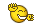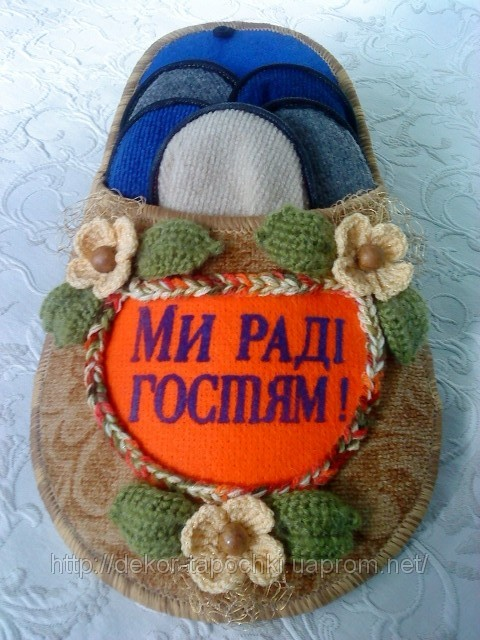Форум - Замок

# Про тапочки...

## Recommended PostsМне проще-мои танцы в обуви не нуждаются )).

##### Share on other sites

Мне проще-мои танцы в обуви не нуждаются )).

Ада, а что это у тебя за танцы такие? :)
##### Share on other sites

Наверное - танцы на животе))))))))))))

##### Share on other sitesОни самые )))).

Хотя там задействованы все части тела )))).

##### Share on other sites

Лег на живот...и никак не пойму - а чешки то зачем)))))))))))))

##### Share on other sites

Лег на живот...и никак не пойму - а чешки то зачем)))))))))))))ну как же - ноги у тебя вверху болтаются??? вот на них надо чешки одеть, чтобы не мерзли )))))

В общем, приеду научу тебя танцу живота )))))

Кстати, я сегодня туфельки себе для вальса купилавсе-таки закрытые, каблук 5 см - удобные, как тапочки, уже опробовала :)

##### Share on other sites

Ага ))) Та я Алеся уже сколько времени и во всех темах все приглашаю и приглашаю, а он все никак... :))))

Тю, ты шо не знаешь шо тут евонная жена тоже постится? )))) Боится шкандалю.

А то я уже пару месяцев хожу вальс танцевать (готовлюсь к поездке в Израиль))))...

Прикидую як ты с Алесем польку-бабочку сбацаете. Бо шо тот вальс? Ну покружились, ну пощупались, та и всех делов. А полька-бабочка до такого безобразия недоступна. Расстояние меж партнёрами))))

так мне ж потрогать и померить надо )))

О! Тепер я бачу що ти щира українка бо як і я поки не помацаю не повірю очам своїм))))))))))

Ага, а вдруг мне партнер на ногу наступит...)))

Тогда оптимум - кирзовые сапоги)))))))

Не Сань, лучше сразу в морду! Извинения то извинениями но больно ведь. Вот в морду для паритету и надоть. ))
##### Share on other sites

Тю, ты шо не знаешь шо тут евонная жена тоже постится? )))) Боится шкандалю.

Аленка мне разрешает :))))

Прикидую як ты с Алесем польку-бабочку сбацаете. Бо шо тот вальс? Ну покружились, ну пощупались, та и всех делов. А полька-бабочка до такого безобразия недоступна. Расстояние меж партнёрами))))

Мы будем фигурный вальс танцевать (я теперь даже знаю, шо это такое) в меееедленном темпе :))))

О! Тепер я бачу що ти щира українка бо як і я поки не помацаю не повірю очам своїм))))))))))

Во-во! )))))

Не Сань, лучше сразу в морду! Извинения то извинениями но больно ведь. Вот в морду для паритету и надоть. ))

та лучше не надо ))) а то ведь не только мне наступают, а и я иногда наступаю :)))
##### Share on other sites

Аленка мне разрешает :))))

Мы будем фигурный вальс танцевать (я теперь даже знаю, шо это такое) в меееедленном темпе :))))

Ага, ты так сексуально описала медленный танец шо ещё вопрос рпзрешит ли тебе Алёнка. )))
##### Share on other sites

Толь...

Ну яка там полька...

Я спец по чуйственным танцам...

Румба, танго, пасодобль...

##### Share on other sites

Ага, ты так сексуально описала медленный танец шо ещё вопрос рпзрешит ли тебе Алёнка. )))Здорово, конечно, что в этом моем описании ты сексуальность увидел )))))

Ну, если не разрешит, значит будем танцевать с Аленкой (она мне обещала)))), а Алесь будет только наблюдать )))

(вообще в фигурном вальсе особо сексуального я ничего не заметила, разве что взглядом если )))))

Толь...

Ну яка там полька...

Я спец по чуйственным танцам...

Румба, танго, пасодобль...а ты попробуй польку чуйственно станцевать )))))

а танго - это да, только один раз пробовала, мало чего поняла, но очень понравилось :))))

##### Share on other sitesа ты попробуй польку чуйственно станцевать )))))

Я чуйственно люблю под такое танцевать ))))

Это школа Соломона Кляра,

Школа бальных танцев, вам говорят.

Две шаги налево, две шаги направо,

Шаг вперед и две назад.

Кавалеры приглашают дамов!

Там, где брошка, там перед.

Две шаги налево, две шаги направо,

Шаг вперед и поворот.

##### Share on other sites

Так ты, Толь, специалист по чечетке?

Ведь именно в семье Кляра она и зародилась...

Детей было 12...а ночной горшок - ОДИН)))

##### Share on other sites

Так ты, Толь, специалист по чечетке?

Ведь именно в семье Кляра она и зародилась...

Детей было 12...а ночной горшок - ОДИН)))

Танцевать не могу, но стэп обожаю. Такое откаблучивать не так то просто. Я имею ввиду профессиональный степ.

Относительно горшка. Так я понял шо строки-

Дамы дамы, помогите Боре,

Помогите Боре вам говорят

Он наделал лужу в коридоре

Шаг вперёд и две назад...

Не добежал сердешный Боря или очереди не дождался?

##### Share on other sites

Не дождался, бедолага)))

##### Share on other sites

Не дождался, бедолага)))

Весьма жизненная и правдивая песня! )))
##### Share on other sites

Дайте и мне тапочку, пожалуссста

##### Share on other sites

Дайте и мне тапочку, пожалуссста

А Вы девочка или мальчик? и какой у Вас размер? )))
##### Share on other sites

Piyava - женского полу, если я правильно поняла!

Выбирайте!И, когда искала тапочки, наткнулась на вот такие, необычные. Ну, это в том случае, если не боитесь... Я бы сама ближе чем на метр к этим тапочкам не приблизилась бы... Но не показать не могу :)##### Share on other sites

Ой, какие прелестные тапочки)))) Мне правда, нужна только одна....

Спасибо милая Орлика, за мышек, и Вам, Фанни, тоже)))))

##### Share on other sites

Ну, как же не за что))))) за проявленное внимание

##### Share on other sites

Ой, какие прелестные тапочки)))) Мне правда, нужна только одна....

На одну ногу что ли ?

## Join the conversation

You can post now and register later. If you have an account, sign in now to post with your account.×   Pasted as rich text.   Paste as plain text instead

Only 75 emoji are allowed.